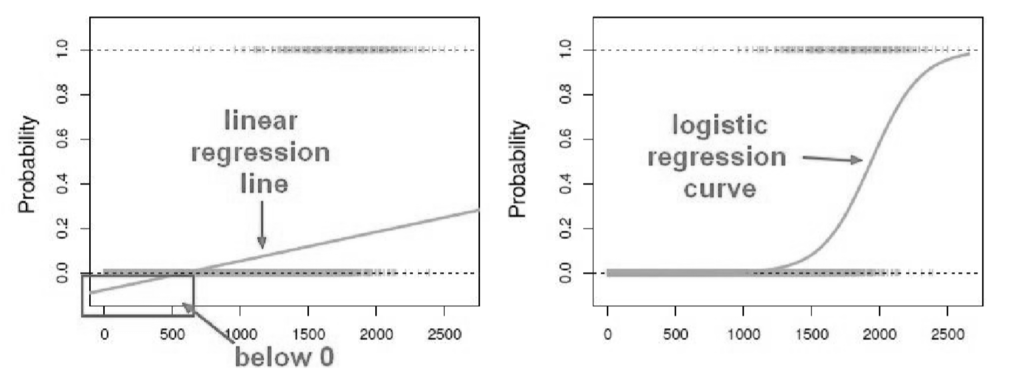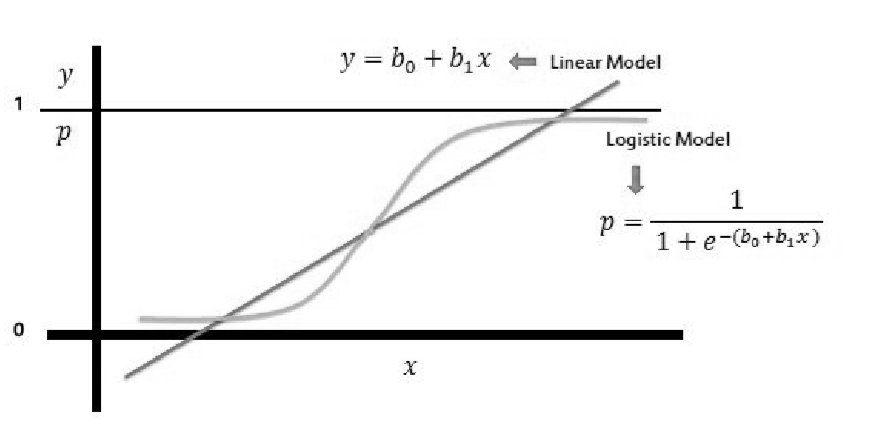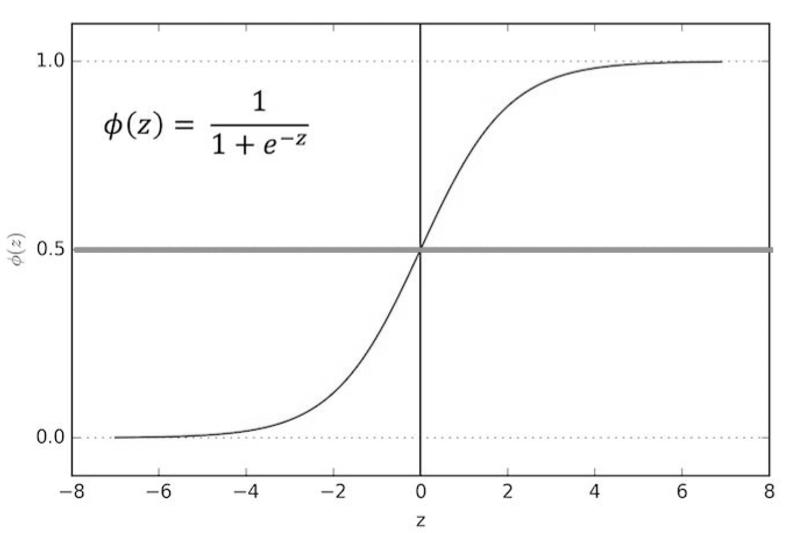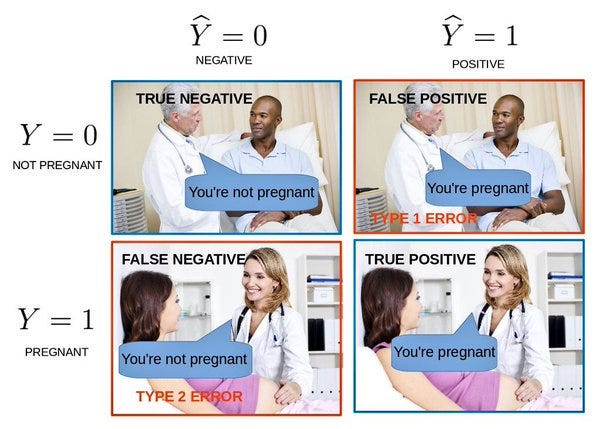# Logistic Regression in Python

In this article, we will learn about Logistic regression and how to implement logistic regression in Python on Titanic Dataset. This will also cover the concepts related to logistic regression and classification in machine learning.

In machine learning and statistics classification is a problem of identifying to which of a set of categories a new observation belongs to based on the training data.

Some examples of classification problems include:

• To check a Spam vs “Hamemail
• Diagnosis of a disease eg. To tell if someone has cancer or not

These all are examples of binary classification meaning we have two classes.

I personally did the below mentioned courses while learning python and machine learning using python, so I hope the readers can use these courses to learn python and data science as well. These courses are pretty amazing and balanced. By balanced I mean correct amount of conceptual and practical content.
linear classifiers in Python — This course is amazing for learning about SVM and logistic regression. So if you want to go deep into such techniques, this is the course which I personally recommend.
Supervised learning in Python — Is another amazing course which one can do to learn about supervised learning techniques in python and their implementation.
Intro to python for Data Science — Is a course for all the beginners who are new to python and want to start off with data science in python. This is the course which I did initially while learning Python.
• So far we have only seen regression problems where we try to predict a continuous value such as the price of the house by drawing a straight line curve
• Using logistic regression we can solve classification problems where we are trying to predict discrete values.
• The convention for binary classification is to have two classes 0 and 1

We can’t use a normal linear regression model on binary groups, it won’t lead to a good fit

Now if this was our training data and we are trying to use linear regression model on it we would get a very bad fit, we would actually end up predicting probabilities less than 0%which doesn’t make any sense,

Instead, we can transform are linear regression curve to a logistic regression curve because our linear regression curve won’t fit our binary group models properly and you can see our logistic regression curve can only go between 0and 1and that is gonna be the key to understand classification using logistic regression curve.Conversion Of Linear To Logistic Regression Curve

### Sigmoid Function

The sigmoid function also known as the logistic function is going to be the key to using logistic regression to perform classification.

• The sigmoid function takes in any value and outputs it to be between 0and 1.

The key thing to notice here is that it doesn’t matter what value of z you put into the logistics or the sigmoid function you’ll always get a value between 0 and 1.

• This means we can take our linear regression solution and place it into the sigmoid function and it looks something like this:Linear Curve in Logistic Regression Curve
• If you take that linear model and place it into a sigmoid function then we are finally able to transform linear regression to logistic model meaning it doesn’t matter whatever the value of linear model output actually is it’s always going to be between 0and 1when you place it into the sigmoid function.

This results in a probability from 0to 1belonging in class 1.

• We can set a cutoff point at 0.5and we can say anything below 0.5results in class 0 and anything above 0.5 belongs to class 1.Logistic Regression Curve with cut-off point

So we are going to transform that 0.5 probability as a cut off point.

### Model evaluation

After we have trained a logistic regression model on some training dataset we can evaluate the model’s performance on some test dataset, we can use confusion matrix to evaluate classification models.

#### Confusion matrix:

The confusion matrix is a table test is often used to describe the performance of the classification model on the test data for which the true values are already known, so we can use a confusion matrix to evaluate a model.

#example: testing the presence of a disease

NO = negative test = False = 0

YES = positive test = True = 1

#### Basic Terms:

• True Positives(TP)= are the cases in which we predicted yes they have the disease and in reality, they do have the disease.
• True Negative(TN)= are the cases in which we predicted no they don’t have the disease and in reality, they don’t have the disease.
• False Positive(FP) = are the cases in which we predicted yes they have the disease and in reality, they don’t have the disease. This is also known as Type 1 Error.
• False Negative(FN)= are the cases in which we predicted no they don’t have the disease and in reality, they do have the disease. This is also known as the Type 2 Error.

#### Accuracy:

how often is it correct?

Accuracy = (TP+TN)/Total

Accuracy = (100+50)/165 = 0.91

#### Misclassification Rate:

how often is it wrong?

MR = (FP+FN)/total

MR = (10+5)/165 = 0.09

This is also called as the Error RateType 1 and Type 2 error

#### Type of Errors:

1. Type 1 Error(False Positive)
2. Type 2 Error(False Negative)

### Types of Logistic Regression

Logistic Regression is basically of 3types-

1. Binary Logistic Regression

The categorical response has only two 2 possible outcomes.

#Example:If your Email is ‘Spam’ or ‘Ham

2. Multinomial Logistic Regression

Three or more categories without ordering.

#Example: Predicting which food is preferred more (Veg, Non-Veg, Vegan)

3. Ordinal Logistic Regression

Three or more categories with ordering.

#Example:Movie rating from 1to 5

### #KeyFeatures

• Logistic regression predicts whether something is True(1)or False(0)instead, predicting something that is continuous like size.
• It has an S-shaped line.
• We can take our Linear Regression Model and convert it into Logistic Regression Model with the help of Sigmoid Function.
• Logistic Regression’s ability to provide probabilities and classify new samples using continuous and discrete measurements makes it a popular machine learning method.

• it doesn’t require high computational power
• is easily interpretable
• is used widely by the data analyst and data scientists.
• is very easy to implement
• it doesn’t require scaling of features
• it provides a probability score for observations.

• while working with Logistic regression you are not able to handle a large number of categorical features/variables.
• it is vulnerable to overfitting
• it cant solve the non-linear problem with the logistic regression model that is why it requires a transformation of non-linear features
• Logistic regression will not perform well with independent(X) variables that are not correlated to the target(Y) variable.

Now let’s go ahead and start to explore an example of Logistic Regression using the famous titanic data set where we try to predict whether or not a passenger survived based off of their features provided to us in our dataset.

### Implementation in Python-

For this portion of the blog, we will be working with the Titanic Data Set from Kaggle.This is a very famous data set and very often is a student’s first step in machine learning!

We’ll be trying to predict a classification- survival or deceased.

Let’s begin our understanding of implementing Logistic Regression in Python for classification.

We’ll use a “semi-cleaned”version of the Titanic data set, if you use the data set hosted directly on Kaggle, you may need to do some additional cleaning not shown in this article.

• Let’s import some libraries to get started!
`import pandas as pdimport numpy as npimport matplotlib.pyplot as pltimport seaborn as sns%matplotlib inline`
• Let’s start by reading in the titanic_train.csv file into a pandas data frame.
`train = pd.read_csv('titanic_train.csv')train.head()`
• Here’s the Data Dictionary, so we can understand the columns info . better:
1. PassengerID-type should be integers
2. Survived-survived or not
3. Pclass-class of Travel of every passenger
4. Name- the name of the passenger
5. Sex -gender
6. Age-age of passengers
7. SibSp -No. of siblings/spouse aboard
8. Parch-No. of parent/child aboard
9. Ticket-Ticket number
10. Fare -what Prices they paid
11. Cabin -cabin number
12. Embarked-the port in which a passenger has embarked.

C -Cherbourg , S -Southhampton , Q -Queenstown

• As we can see here, the ship was very big, so there must be a lot of people there, let’s see how many people:
`train.count()`

Ok, we can see 891 total. There are some null values for some columns, later we are going to deal with that.

• information on the dataset
`train.info()`
• Getting useful details from the data frame
`train.describe()`

### Exploratory Data Analysis

Let’s begin some exploratory data analysis!

There is another amazing course to learn about how to start preprocessing data for any ML project — Pre-processing for ML in python . This is amazing for learning how to start with any data science project, as pre-processing is one of the most important and initial steps when solving and ML problem.

We’ll start by checking out missing data from our data frame and replacing it with useful data.

#### Missing Data

We can use seaborn to create a simple heatmap to see where we are missing data!

`sns.heatmap(train.isnull(),yticklabels=False,cbar=False,cmap='viridis')`

Roughly 20 percent of the Age data is missing. The proportion of Age missing is likely small enough for reasonable replacement with some form of imputation. Looking at the Cabin column, it looks like we are just missing too much of that data to do something useful with at a basic level. We’ll probably drop this later, or change it to another feature like “Cabin Known: 1 or 0”

### Data Visualizations

Let’s continue on by visualizing some more of the data!

Now in this project I have used Seaborn library for data viz. The best resource out there to learn Seaborn is Data Visualization with Seaborn. Do check out this one, if you want to become a master in data visualization.
`#count-plot of people survided sns.set_style('whitegrid')sns.countplot(x='Survived', hue='Sex', data=train, palette='RdBu_r')`
• after looking at this graph we can tell that the people who did not survive were much more likely to be male and people who did survive were almost like twice as likely to be female.
`#no. of people who survived according to their Passenger Classsns.set_style('whitegrid')sns.countplot(x='Survived', hue='Pclass', data=train)`
• after looking at this we can tell that people who did not survive were more likely to be belonging to third class i.e the lowest class, the cheapest to get on to and people who did survive were more towards belonging to higher classes.
`#distribution plot of age of the peoplesns.distplot(train['Age'].dropna(), kde=False, bins=30, color='Green')`
• The average age group of people to survive is somewhere between 20 to 30and as older you get lesser chances of you to have on board.
`#countplot of the people having siblings or spoucesns.countplot(x='SibSp',data=train)`
• looking at this plot we can directly tell that most people on board did not have either children, siblings or spouse on board and the second most popular option is 1which is more likely to be spouse. We have a lot of single people on board, they don’t have spouse or children.
`#distribution plot of the ticket faretrain['Fare'].hist(color='green',bins=40,figsize=(8,4))`
• It looks like most of the purchase prices are between 0 and50, which actually makes sense tickets are more distributed towards cheaper fare prices because most passengers are in cheaper third class.

### Data Cleaning

We want to fill in missing age data instead of just dropping the missing age data rows. One way to do this is by filling in the mean age of all the passengers. However, we can be smarter about this and check the average age by passenger class.

Cleaning data is another important step for any data science project. Hence there is another amazing course for learning it properly i.e Data Cleaning in Python . Do check this out as well as all these steps are really important when doing any data science project.

For example:

`#boxplot with age on y-axis and Passenger class on x-axis.plt.figure(figsize=(12, 7))sns.boxplot(x='Pclass',y='Age',data=train,palette='winter')`

We can see the wealthier passengers in the higher classes tend to be older, which makes sense. We’ll use these average age values to impute based on Pclass for Age.

`def impute_age(cols):    Age = cols    Pclass = cols        if pd.isnull(Age):`
`if Pclass == 1:            return 37`
`elif Pclass == 2:            return 29`
`else:            return 24`
`else:        return Age`

Now apply that function!

`train['Age'] = train[['Age','Pclass']].apply(impute_age,axis=1)`

Now let’s check that heatmap again!

`sns.heatmap(train.isnull(),yticklabels=False,cbar=False,cmap='viridis')`

Now let us go ahead and drop the Cabin column and the row in Embarked that is NaN.

`train.drop(‘Cabin’,axis=1,inplace=True)train.dropna(inplace=True)train.head()`

### Converting Categorical Features

We’ll need to convert categorical features to dummy variables using pandas! Otherwise, our machine learning algorithm won’t be able to directly take in those features as inputs.

`sex = pd.get_dummies(train['Sex'],drop_first=True)embark = pd.get_dummies(train['Embarked'],drop_first=True)`
`#drop the sex,embarked,name and tickets columnstrain.drop(['Sex','Embarked','Name','Ticket'],axis=1,inplace=True)`
`#concatenate new sex and embark column to our train dataframetrain = pd.concat([train,sex,embark],axis=1)`
`#check the head of dataframetrain.head()`

Now our data is ready for our model!

### Building a Logistic Regression model

Let’s start by splitting our data into a training set and test set(there is another test.csv file that you can play around with in case you want to use all this data for training).

### Train Test Split

• X will contain all the features and y will contain the target variable
`from sklearn.model_selection import train_test_split`
`X_train, X_test, y_train, y_test = train_test_split(train.drop('Survived',axis=1),            train['Survived'], test_size=0.30,             random_state=101)`
• Here y is the actual data which we are going to predict, everything else is going to be the features(x).
• Set the text size to 30 percent and you don’t actually have to set your random state but this is put so if you want your result to match mines exactly.
• We will use train_test_split from the cross_validation module to split our data. 70%of the data will be training data and %30 will be testing data.
• You can read more about Train_Test_Split

### Training and Predicting

• Let’s use Logistic Regression to train the model
`from sklearn.linear_model import LogisticRegression`
`#create an instance and fit the model logmodel = LogisticRegression()logmodel.fit(X_train, y_train)`
• We start by importing the LogisticRegression package from the Linear model family.
• Then create an instance of the logistic regression model and call it log model and then fit the model on the training dataset.
• Let’s see how accurate is our model for predictions
`#predictionsPredictions = logmodel.predict(X_test)`
• Now we call some predictions based on the X_test dataset.

### Model Evaluation

• We can check precision, recall, f1-score using classification report and also see how accurate is our model for predictions:
`from sklearn.metrics import classification_report`
`print(classification_report(y_test,predictions))`

->We got 81% accuracy which is not bad at all.

Let us now see the confusion matrix:

To evaluate our model for some specific values, it can be directly done from our confusion matrix.

`from sklearn.metrices import confusion_matrix`
`print(confusion_matrix(y_test, predictions))`

From our confusion matrix we conclude that:

• True positive: 148(We predicted a positive result and it was positive)
• True negative: 68(We predicted a negative result and it was negative)
• False positive: 15(We predicted a positive result and it was negative)
• False negative: 36(We predicted a negative result and it was positive)

Accuracy = (TP+TN)/total

Accuracy = (148+68)/267 ~ 81%

Error Rate = (FP+FN)/total

Error rate = (36+15)/267 ~19%

### Conclusion:

• We now know what the logistic function is and how it is used in logistic regression.
• The homogeneity of variance does not need to be always TRUE for the Logistic Regression model.
• Logistic Regression uses maximum likelihood estimation (MLE) rather than ordinary least squares (OLS) to estimate the parameters, therefore its predictions depend upon large-sample approximations.
• Logistic Regression does not assume a linear relationship between the dependent and the independent variables, but it will assume a linear relationship between the logic of the explanatory variables and the response.

In our tutorial, we have covered a lot of details about Logistic Regression. You have learned what Logistic Regression is, how to build Logistic regression models, how to visualize the results, how to deal with missing data and some of the theoretical background information.

Also, we have covered some basic concepts such as the sigmoid function, confusion matrix, exploratory data analysis, Converting Categorical Features, building logistic regression model.

We still can improve our model, but this tutorial is intended to show how we can do some exploratory analysis, clean up data, and implement logistic regression in python.

Hope you all liked this article. Do like and share this article with your peers. For any doubts feel free to comment down below.

MRINAL WALIA has helped me a lot in my journey in learning data science in python. So you folks can follow us on Github and our other social profiles. Feel free to bug me or this guy for any doubts regarding data science in python.